# Direct Torque Fuzzy Controlled Drive for Multi-phase IPMSM Based on SVM Technique

Direct Torque Fuzzy Controlled Drive for Multi-phase IPMSM Based on SVM Technique

Electrical Engineering Department, Hassiba Benbouali University, Laboratoire Génie Electrique et Energies Renouvelables (LGEER), Chlef 02000, Algeria

ENP Maurice Audin, Oran 31000, Algeria

Corresponding Author Email:
f.mehedi@univ-chlef.dz
Page:
259-266
|
DOI:
https://doi.org/10.18280/jesa.530213
8 December 2019
|
Accepted:
19 February 2020
|
Published:
20 May 2020
| Citation

OPEN ACCESS

Abstract:

This work presents an improved Direct Torque Control (DTC) method for a 5-phase interior permanent magnet synchronous motor (5P-IPMSM) based on space vector modulation (SVM) and fuzzy logic (FL). In this proposed DTC control, we replaced the traditional proportional-integral (PI) by the FL controller. The main goal is to improve system performance by minimizing the ripple of the stator flux and the torque ripples. The proposed control technique applied on the 5P-IPMSM is verified by Matlab/Simulink software followed by an analyzed and compared to verify the effectiveness of the proposed method. Many improvements have been made to the stator flux ripples, torque ripples, rise time and the robustness to disturbance.

Keywords:

direct torque control, stator flux ripple, fuzzy logic controller, multi-phase interior permanent magnet synchronous motor, torque ripple, space vector modulation

1. Introduction

Multiphase machines (MPMs) have useful properties compared to a traditional three-phase system . Among them, for the same power rating, the phase currents are much smaller in MPMs and characterized by an inherent fault tolerance that can be improved [2, 3]. As for the application areas, they are many and varied, such as automobiles and the wind energy conversion system [4, 5].

Direct torque control (DTC) is the most important method used nowadays. The DTC strategy was first proposed for the induction motor (IM) in 1985 by Takahashi et al. . The basic idea of this technique is a direct command of the stator flux and torque of IM without any pulse width modulation (PWM) strategy and proportional-integral (PI) controllers. However, this method is a simple structure compared to the field-oriented control (FOC) strategy. In this method, the switching table is used to control the inverter. On the other hand, the DTC method has many advantages, the fast dynamic response, lower parameter dependency, and reliability. But, the principal drawbacks, is the stator flux and torque ripples [7, 8].

A DTC control scheme was proposed to control permanent synchrone motor (PMSM) . A DTC technique was designed to regulate the torque of the doubly fed induction machine (DFIM) . The authors  propose a DTC strategy based on the neural controller. In this proposed DTC control, the switching table is replaced by artificial neural networks (ANNs) controller. The DTC control is proposed to command the IM drive using a five-level neutral point clamped (NPC) inverter . This proposed technique minimized the total harmonic distortion (THD) of voltage and torque ripple compared to classical DTC strategy. The DTC method was proposed based on space vector modulation (SVM) to command IM drive by using two PI controllers .

DTC control scheme was proposed based on second order sliding mode control (SOSMC) to command the DFIG-based wind power . The switching table of DTC control is replaced by the fuzzy logic controller (FLC) . This proposed strategy is simple scheme and minimize the ripples in torque and stator flux. The authors propose a four-level DTC strategy with the ANN controller by using neural PI controller of speed . The switching table of DTC is replaced by ANN controller and PI controller of speed by using fuzzy controller .

The direct flux and torque control of speed sensorless five-phase interior permanent magnet synchronous motor (5P-IPMSM) based on adaptive sliding mode control (ASMC) is presented . A new fault tolerant drives for a 5P-IPMSM to achieve the MTPA operation (maximum torque per ampere) under open-circuit faults . On the other hand, the 5P-IPMSM is an MPM and reduces the electromagnetic torque compared to the classical PMSM. The DTC strategy of 5P-IPMSM based on adaptive input-output feedback linearization (AIOFL) is presented . A SOSMC technique was proposed to control the two 5P-IPMSM drives . FLC technique and sliding mode control (SMC) control are combined to regulate the torque and speed of 5P-IPMSM . The author proposes a comparison between classical DTC and DTC with SVM technique for five-phase induction motor .

In this work, we propose a new DTC method based on the FLC technique named DTC-SVM-Fuzzy. The original contribution is the application of the intelligent control in the DTC method by using SVM technique and simulation investigation of this new method. The main advantages of the DTC-SVM-Fuzzy method are the simplicity of implementation and minimizing the ripple of the stator flux and the torque ripples compared to a traditional DTC system and DTC-SVM with PI controller of the 5P-IPMSM.

2. Conventional DTC Strategy

The principle of DTC method is presented in detail in the papers [6-18]. To learn DTC strategy, we review some of the equations of 5-phase IPMSM. The electromagnetic torque is written in terms of current and stator flux as :

${{T}_{e}}=\frac{5}{2}P({{\Phi }_{\alpha }}{{i}_{\beta }}-{{\Phi }_{\beta }}{{i}_{\alpha }})$      (1)

The switching states of the inverter are determined by flux and torque errors, as shown in Eq. (2):

\left\{ \begin{align} & \Delta {{T}_{e}}={{T}_{e}}^{*}-{{T}_{e}} \\ & \Delta {{\Phi }_{s}}={{\Phi }_{s}}^{*}-{{\Phi }_{s}} \\ \end{align} \right.      (2)

where,

Te* : Reference torque.

Φs* : Reference flux.

Using the quantities of Concordia, the amplitude of the stator flux is expressed by:

${{\Phi }_{s}}=\sqrt{{{\Phi }_{\alpha }}^{2}+{{\Phi }_{\beta }}^{2}}$       (3)

And position of the stator flux:

${{\theta }_{s}}={{\tan }^{-1}}\frac{{{\Phi }_{\beta }}}{{{\Phi }_{\alpha }}}$       (4)

The DTC-ST control scheme contains three control loops: the control loop for the electromagnetic torque in which the hysteresis comparator, as well as the control loop for the magnitude of the flux has a hysteresis comparator, while the speed control loop for the motor has a PI regulator. In addition, the classic DTC is a simple control scheme and easy to implement compared to vector control. However, this strategy gives more ripples in stator flux and electromagnetic torque, due to the variable switching frequency.

Listwan and Pieńkowski  proposed a new switching table for classical DTC of the five-phase IPMSM drives; this proposed switching table is given in Table 1. On the other hand, the modeling of a 5-phase IPMSM is expressed in a rotary frame d-q-x-y as [25, 26]:

The state equation of stator voltage:

\left\{ \begin{align} & v_{ds}^{{}}={{R}_{s}}i_{ds}^{{}}+\frac{d}{dt}\Phi _{ds}^{{}}-{{w}_{r}}\Phi _{qs}^{{}} \\ & v_{qs}^{{}}={{R}_{s}}i_{qs}^{{}}+\frac{d}{dt}\Phi _{qs}^{{}}+{{w}_{r}}{{\Phi }_{ds}} \\ & v_{xs}^{{}}={{R}_{s}}i_{xs}^{{}}+\frac{d}{dt}\Phi _{xs}^{{}} \\ & v_{ys}^{{}}={{R}_{s}}i_{ys}^{{}}+\frac{d}{dt}\Phi _{ys}^{{}} \\ & v_{0s}^{{}}={{R}_{s}}i_{0s}^{{}}+\frac{d}{dt}\Phi _{s0}^{{}} \\ \end{align} \right.       (5)

Table 1. Switching table for conventional DTC

 dɸ +1 0 dT +1 0 -1 +1 0 -1 S1 24 0 17 14 31 7 S2 28 31 25 6 0 3 S3 12 0 24 7 31 19 S4 14 31 28 3 0 17 S5 6 0 12 19 31 25 S6 7 31 14 17 0 24 S7 3 0 6 25 31 28 S8 19 31 7 24 0 12 S9 17 0 3 28 31 14 S10 25 31 19 12 0 6

\left\{ \begin{align} & \Phi _{ds}^{{}}={{L}_{d}}i_{ds}^{{}}+{{\varphi }_{f}} \\ & \Phi _{qs}^{{}}={{L}_{q}}i_{qs}^{{}} \\ & \Phi _{xs}^{{}}={{L}_{ls}}i_{xs}^{{}} \\ & \Phi _{ys}^{{}}={{L}_{ls}}i_{ys}^{{}} \\ & \Phi _{s0}^{{}}={{L}_{ls}}i_{0s}^{{}} \\ \end{align} \right.    (6)

The electromagnetic torque is expressed as follow:

${{T}_{e}}=\frac{5}{2}P(({{L}_{d}}-{{L}_{q}}){{i}_{ds}}{{i}_{qs}}+{{\varphi }_{f}}{{i}_{qs}})$      (7)

The fundamental equation of dynamics is:

${{J}_{m}}\frac{d{{w}_{r}}}{dt}={{p}_{{}}}{{T}_{e}}-{{p}_{{}}}{{T}_{r}}-{{f}_{m}}{{w}_{r}}$      (8)

3. DTC-SVM with PI Controller

To improve classic DTC performance of the five-phase IPMSM, we propose using a new DTC control scheme with SVM technique. However, the DTC-SVM method is a simple scheme and reduces stator flux ripple and electromagnetic torque ripple compared to the classic DTC and vector control.

A 5-phase vector provides a total of thirty-two spatial vectors, of which thirty are active vectors and two are zero vectors. The 30 vectors extend over 360 degrees in a two-dimensional plane, forming a decagon with ten sectors of 36 degrees each. The 5-phase SVM can be applied using a two or four vector method. The active switching vectors are divided in to three groups; large, medium and small switching vectors. The active switching vectors are divided in to 3 groups: small, large and medium switching vectors. Figure 1 (a) and Figure 1 (b) illustrate the active vectors for α-β frame and x-y frame, respectively [27, 28].

## a.png(a)

## b.png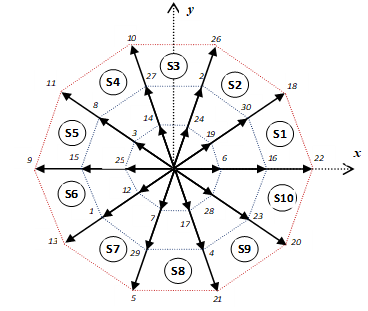(b)

Figure 1. The voltage space vectors and the switching states of the five-phase inverter in: (a) α-β frame, (b) x-y frame

The expression for switching time (ST) using the four-vector method is given as follows:

$V_{s}^{*}{{T}_{s}}=V_{al}^{{}}T_{al}^{{}}+V_{bl}^{{}}T_{bl}^{{}}+V_{am}^{{}}T_{am}^{{}}+V_{bm}^{{}}T_{bm}^{{}}$      (9)

\left\{ \begin{align} & \left| V_{al}^{{}} \right|=\left| V_{bl}^{{}} \right|=\left| V_{l}^{{}} \right|=\frac{2}{5}{{V}_{dc}}2\cos \left( \frac{\pi }{5} \right) \\ & \left| V_{am}^{{}} \right|=\left| V_{bm}^{{}} \right|=\left| V_{m}^{{}} \right|=\frac{2}{5}{{V}_{dc}} \\ \end{align} \right.      (10)

$\frac{T_{al}^{{}}}{T_{am}^{{}}}=\frac{T_{bl}^{{}}}{T_{bm}^{{}}}=\frac{\left| V_{l}^{{}} \right|}{\left| V_{m}^{{}} \right|}=\tau =1.618$      (11)

We find the equation for the ST by solving equation (9), (10) and (11). These are as follows:

\left\{ \begin{align} & T_{am}^{{}}=\frac{\left| 0.2764{{V}_{ref}} \right|\sin \left( k\pi /5-\theta \right)}{\left| {{V}_{m}} \right|\sin \left( \pi /5 \right)}T_{s}^{{}} \\ & T_{al}^{{}}=\frac{\left| 0.7236{{V}_{ref}} \right|\sin \left( k\pi /5-\theta \right)}{\left| {{V}_{l}} \right|\sin \left( \pi /5 \right)}T_{s}^{{}} \\ & T_{bm}^{{}}=\frac{\left| 0.2764{{V}_{ref}} \right|\sin \left( \theta -\left( k-1 \right)\pi /5 \right)}{\left| {{V}_{m}} \right|\sin \left( \pi /5 \right)}T_{s}^{{}} \\ & T_{bl}^{{}}=\frac{\left| 0.2764{{V}_{ref}} \right|\sin \left( \theta -\left( k-1 \right)\pi /5 \right)}{\left| {{V}_{l}} \right|\sin \left( \pi /5 \right)}T_{s}^{{}} \\ & T_{0}^{{}}=T_{s}^{{}}-\left( T_{am}^{{}}+T_{al}^{{}}+T_{bm}^{{}}+T_{bl}^{{}} \right) \\ \end{align} \right.       (12)

where, Ts switching period; Tam, Tbm the ST of medium voltage vectors; T0 the ST of zero voltage vectors; Tal, Tbl the ST of long voltage vectors; θ the angle of position of reference voltage vector; Vref reference voltage vector; k number of sector.

To optimize the performance of the DTC strategy and the application of a fixed switching frequency, the hysteresis comparator in a DTC traditional is compensated by PI stator flux and torque comparators. The switching table is also replaced by an SVM Technique, as shown in Figure 2.

## 2.pngFigure 2. Diagram of DTC-SVM control applied for five-phase IPMSM

4. Fuzzy Logic DTC-SVM for Five-Phase IPMSM

Figure 5 represents the technique of the direct torque fuzzy controller (DTFC). The traditional PI controllers for torque and flux have been replaced by two FLC. The Te* and Φs* are compared with the values obtained in the Te and Φs estimator. The errors are sent to the DTFC control system. The membership function (MF) of the combined input and output variables is generally determined by a common universe of discourse. The FLC is based on the following elements: a fuzzification interface, an inference mechanism, a bases rule and a defuzzification interface [29, 30].

The if-then rules to FLC for speed control will be 25 rules. Figure 3 (a), (b) and (c) shows MFs of input variables Ew, dEw and output variable respectively (traditional triangular shapes). The MFs is divided into 5 fuzzy. Table 2 shows the 25 fuzzy control rules.

## 3.png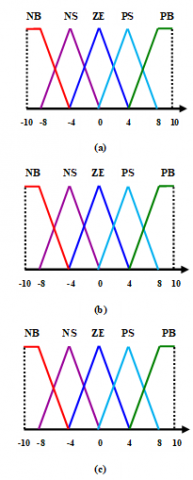Figure 3. Speed regulator variables membership: (a) Ew, (b) dEw, (c) output

Table 2. FLC rule base for speed control

 Ew NS PB ZE NB PS dEw NB ZE PB PB ZE PB PB NB ZE NB NB ZE ZE ZE NS ZE PS ZE PS NS ZE NS NS ZE NS ZE PS PS ZE PS

The fuzzy labels used in this method are positive small (PS), equal zero (ZE), positive big (PB), negative big (NB) and negative small (NS).

The same steps used for the conception of the speed controller (FLC) will be repeated for the torque and flux controller as shown in Figure 4, only we have: EΦ= Φs *- Φs  for the first fuzzy controller of flux Φs and for the second fuzzy controller of torque ET= Te*- Te  for the first fuzzy controller of torque Te.

We represent the input/output variables by MF, as show in Figure 4, each one divided into 3 fuzzy. Table 3 shows the 9 fuzzy control rules and bestow the change of the FLC output in terms of two inputs EΦ,T and dEΦ,T.

## 4.pngFigure 4. Torque and flux regulator variables membership: (a) EΦ,T, (b) dEΦ,T, (c) output

Table 3. The rule base for controlling the torque and flux

 EΦ,T P Z N dEΦ,T N Z N N P P P Z Z P Z N

The MFs is also assigned with three fuzzy sets: Z (zero), N (negative), P (positive).

The bloc diagram of the DTC-SVM fuzzy for five-phase IPMSM is given by Figure 5.

## 5.png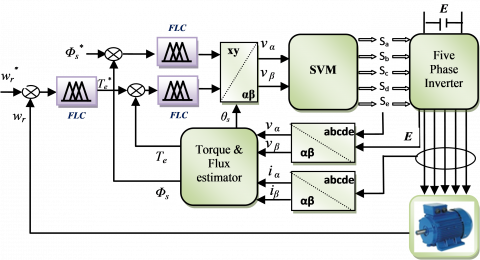Figure 5. Block diagram of the five-phase IPMSM with DTC-SVM-Fuzzy

5. Simulation Results and Discussion

To verify the method proposed in this work, Matlab/Simulink software based simulations were performed. The parameters of the 5-phase IPMSM are as follows: Rs = 0.7 Ω, Jm = 0.0025 Kg/m2, fm = 0.005 Nm/rad.s-1, Ld = 0.0018 H, Lq = 0.0042 H, P=2, ϕf=0.5 web.

Three control strategies: DTC-ST, DTC-SVM and DTC-SVM-fuzzy are simulated and compared to reference tracking, sensitivity to load torque change, torque and stator flux ripples, and robustness against variations in machine parameters.

5.1 Reference tracking test

Figure 6 show the performance of the 5-phase IPMSM under change in the Trfrom 0 Nm to 10 Nm at t = 0.3s, after that, the Tr is reduced to 0 Nm at t = 0.6 s. The reference speed is set at 100 to -100 rad/s at t=0.9s. This figure shows that the effect of the Tris very evident on the speed curve of the DTC with the PI controller, while the effects are negligible for the DTC with FLC (see Figure 10). We can see that the latter has an almost ideal speed disturbance rejection (about 2%).

Figure 7 presents the response of torque with Tr application. A comparison of the results obtained indicates that the DTC-SVM- Fuzzy method reduced torque ripple by 68% compared to traditional DTC and by 20% compared to DTC-SVM (see Figure 11). These results confirm that the torque ripple in the proposed method has considerably decreased. A comparative study is provided in Table 4.

Figure 8 presents the response of the stator flux for these three methods. It is obvious that DTC-SVM-Fuzzy method can improve steady and dynamic performances of the system and decrease flux ripple (see Figure 12). Figure 9 (a) presents the stator flux loci for traditional DTC. The stator flux locus with the DTC-SVM diagram is shown in Figure 9 (b) and with the DTC-SVM-Fuzzy diagram is shown in Figure 9 (c). In Figure 9 (c) the Lucas of stator flux is improved with very low ripple as compared with classical DTC.

## 6.png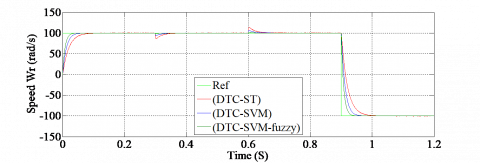Figure 6. Rotor speed response of the five-phase IPMSM controlled by classical DTC, DTC-SVM and DTC-SVM-fuzzy

## 7.png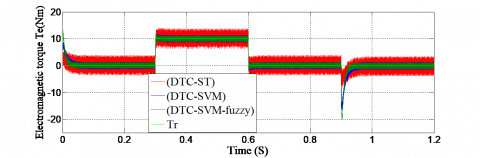Figure 7. Torque response

## 8.pngFigure 8. Stator flux response

## 9a.png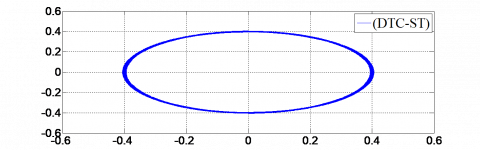(a)

## 9b.png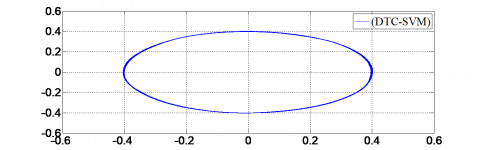(b)

## 9c.png(c)

Figure 9. Stator flux circular trajectory in α-β axis: (a) Classical DTC, (b) DTC-SVM, (c) DTC-SVM-fuzzy

## 10.png(a)                                        (b)

Figure 10. Zoom in the rotor speed response: (a) When start-up, (b) When applying the load torque

## 11.pngFigure 11. Torque response (Zoom)

## 12.pngFigure 12. Stator flux (Zoom)

Table 4. Amplitude of ripples

 Traditional DTC DTC-SVM DTC-SVM-fuzzy Te ripples (Nm) 6,2-13,6  (7,4 Nm) 8,5-11,4  (2,9 Nm) 8,9-11,1  (2,2 Nm) Φs ripples (Wb) 0,393-0,407  (0,014 Wb) 0,396-0,405 (0,009 Wb) 0,398-0,403  (0,005 Wb)

5.2 Robustness test

To study the robustness of the proposed method, the nominal value of the stator resistance Rs and the inertia variation Jm is increased by 100%, Ld and Lq we reduce them by 50%. Simulation results are shown in Figures 13-18. These variations have a clear effect on the rotor speed, torque and stator flux curves and the effect seems more important to DTC-ST and DTC-SVM control scheme compared to DTC-SVM-fuzzy (see Figures 16-18). A comparative study is provided in Table 5. We conclude the FLC that they are more robust to this parameter variation than a PI controller.

## 13.pngFigure 13. Rotor speed response of the five-phase IPMSM controlled by classical DTC, DTC-SVM and DTC-SVM-fuzzy

## 14.pngFigure 14. Torque response

## 15.pngFigure 15. Stator flux response

## 16.png(a)                                        (b)

Figure 16. Zoom in the rotor speed response: (a) When start-up, (b) When applying the load torque

## 17.pngFigure 17. Torque response (Zoom)

## 18.pngFigure 18. Stator flux (Zoom)

Table 5. Amplitude of ripples

 Traditional DTC DTC-SVM DTC-SVM-fuzzy Te ripples (Nm) 4,3-16  (11,7 Nm) 7,9-11,9  (4 Nm) 8,8-11,2 (2,4 Nm) Φs ripples (Wb) 0,389-0,411  (0,022 Wb) 0,392-0,407 (0,015 Wb) 0,397-0,404 (0,007 Wb)
6. Conclusion

In this work, a DTFC of the 5P-IPMSM based on SVM was proposed to improve the performance of the DTC technique. Simulation results achieved by the proposed method of DTC-SVM with FLC confirm a considerable reduction in ripples of the stator flux, torque ripples and the robustness to the system compared to a traditional DTC system and DTC-SVM with PI controller.

For future works, it will address the experimental validation of the proposed method. Next, it is interesting to extend the DTC-SVM with type-2 fuzzy algorithm add an observer for speed estimation.

Nomenclature
 DTC Direct Torque Control IPMSM Interior Permanent Magnet Synchronous Motor FLC Fuzzy Logic Controller PI Proportional-Integral SVM Space Vector Modulation fm Viscous damping Jm Inertia moment Ld , Lq d and q axis stator inductance is Stator currents Rs Stator resistance Lx, Ly x and y axis stator inductance p Number of pairs poles vs Stator voltages Greek symbols θr Electrical angle Te Electromagnetic torque Tr Load torque ϕf Magnetic flux θs Position of the stator flux Φs Stator flux
Appendix

1. Block diagram of the FLC

The block diagram of FL controller is shown in Figure 19.

## 19.pngFigure 19. Block diagram of the FL controller

2. The coefficients of the FLC stator flux, torque and speed controllers

Table 6 shows the constants values of the stator flux, torque and speed FL controller algorithm gains.

Table 6. FL controller gains

 Stator flux Torque Speed K1 K2 K3 K1 K2 K3 K1 K2 K3 200 0.01 50 150 0.01 70 70 0.4 170

The Table 7 shows the parameters of fuzzy controller.

Table 7. Parameters of fuzzy controller

 Fis type Mamdani And method Min Or method Max Implication Min Aggregation Max Defuzzification Centroid
References

 Catuogno, G.R., Garcia, G.O., Leidhold, R., Guazzi, G. (2016). Fault tolerant control in six-phase PMSM under four open-circuits fault conditions. IEEE International Conference on Industrial Electronics, Ischia, Italy. http://dx.doi.org/10.1109/IECON.2016.7793390

 Rangari, S.C., Suryawanshi, H.M, Renge, M. (2018). New fault-tolerant control strategy of five-phase induction motor with four-phase and three-phase modes of operation. Electronics, 7(9): 159-174. http://dx.doi.org/10.3390/electronics7090159

 Hosseyni, A., Trabelsi, R., Mimouni, M.F., Iqbal, A. (2018). Fault tolerant vector controlled five-phase permanent magnet synchronous motor drive with an open phase. IEEE International Multi-Conference on Systems, Signals & Devices (SSD), Hammamet, Tunisia. http://dx.doi.org/10.1109/SSD.2018.8570487

 Salehifar, M., Arashloo, R.S., Eguilaz, M.M., Sala, V., Romeral, L. (2015). Observer-based open transistor fault diagnosis and fault-tolerant control of five-phase permanent magnet motor drive for application in electric vehicles. IEEE IET Power Electronics, 8(1): 76-87. http://dx.doi.org/10.1049/iet-pel.2013.0949

 Bounadja, E., Boudjema, Z., Djahbar, A. (2019). A new DPC-SVM for matrix converter used in wind energy conversion system based on multiphase permanent magnet synchronous generator. Iranian Journal of Electrical and Electronic Engineering (IJEEE), 15(3): 352-363. https://doi.org/10.22068/IJEEE.15.3.352

 Takahashi, I., Noguchi, T. (1986). A new quick-response and high-efficiency control strategy of an induction motor. IEEE Transaction Industry Applications, IA-22 (1): 820-827. http://dx.doi.org/10.1109/TIA.1986.4504799

 Alsofyani, I.M., Idris, N.R.N. (2016). Look-up table-based DTC of induction machines with improved flux regulation and extended kalman filter state estimator at low speed operation. IEEE Transactions on Industrial Informatics, 12(4): 1421-1425. https://doi.org/10.1109/TII.2016.2571682

 Ammar, A., Bourek, A. (2017). Nonlinear SVM-DTC for induction motor drive using input–output feedback linearization and high order sliding mode control. ISA Trans, 67(1): 428-442. http://dx.doi.org/10.1016/j.isatra.2017.01.010

 Abdul Kadir, M.N.S., Mekhilef, S., Ping, H.W. (2007). Direct torque control permanent magnet synchronous motor drive with asymmetrical multilevel inverter supply. IEEE 7th International Conference on Power Electronics, Daegu, South Korea. http://dx.doi.org/10.1109/ICPE.2007.4692567

 Aghasi, S., Khaburi, D.A., Faraji, V., Behnia, H. (2012). A comparative study on predictive and ISVM direct torque control methods for a doubly fed induction machine fed by an indirect matrix converter. Iranian Journal of Electrical & Electronic Engineering (IJEEE), 8(2): 138-145.

 Benbouhenni, H., Taleb, R. (2017). Commande directe du couple neuronale à 6 secteurs de la machine asynchrone alimentée par onduleur NPC à sept niveaux. Revue Proceedings of Engineering and Technology (PET), 14: 137-142.

 Benbouhenni, H., Taleb, R., Chabni, F. (2017). Etude comparative entre la commande DTC trois niveaux et la commande DTC cinq niveaux de la machine asynchrone. Revue Proceedings of Engineering and Technology (PET), 14: 143-149.

 Raj, M.S., Saravanan, T., Srinivasan, Y., Srinivasan, Y. (2014). A modified direct torque control of induction motor using space vector modulation technique. Middle-East Journal of Scientific Research, 20(11): 1572-1574. https://doi.org/10.5829/idosi.mejsr.2014.20.11.114174

 Boudjema, Z., Taleb, R., Djerriri, V. Yahdou, A. (2017). A novel direct torque control using second order continuous sliding mode of a doubly fed induction generator for a wind energy conversion system. Turkish Journal of Electrical Engineering & Computer Sciences, 25(11): 965-975. http://dx.doi.org/10.3906/elk-1510-89

 Gao, S.W., Wang, Y.H., Cai, Y., Zhang, C. (2010). Research on reducing torque ripple of DTC fuzzy logic-based. 2nd International Conference on Advanced Computer Control, Shenyang, China. http://dx.doi.org/10.1109/ICACC.2010.5486721

 Benbouhenni, H. (2019). Four-level direct torque control of permanent magnet synchronous motor based on neural networks with regulation speed using neural PI controller. Majlesi Journal of Mechatronic Systems, 8(4): 965-975.

 Benbouhenni, H. (2018). Seven-level direct torque control of induction motor based on artificial neural networks with regulation speed using fuzzy PI controller. Iranian Journal of Electrical and Electronic Engineering, 14(1): 85-94. http://dx.doi.org/10.22068/IJEEE.14.1.85

 Shishehgar, J., Soltani, J., Tabrizchi, A.M., Abjadi, N.R. (2014). Direct torque and flux control of speed sensorless five-phase interior permanent magnet motor based on adaptive sliding mode control. The 5th Annual International Power Electronics, Drive Systems and Technologies Conference (PEDSTC 2014), Tehran. http://dx.doi.org/10.1109/PEDSTC.2014.6799340

 Chen, Q., Gu, L., Liu, G. (2019). Extension of space-vector-signal-injection based MTPA control into SVPWM fault-tolerant operation for five-phase IPMSM. IEEE Transactions on Industrial Electronics. http://dx.doi.org/10.1109/TIE.2019.2944066

 Tabrizchi, A.M., Soltani, J., Shishehgar, J., Abjadi, N.R. (2019). Direct torque control of speed sensorless five-phase IPMSM based on adaptive input-output feedback linearization. The 5th Annual International Power Electronics, Drive Systems and Technologies Conference (PEDSTC 2014), Tehran. http://dx.doi.org/10.1109/PEDSTC.2014.6799341

 Mehedi, F., Nezli, L., Mahmoudi, M.O., Boudjema, Z. (2017). Second order sliding mode control of two series-connected multi-phase permanent magnet synchronous motor. The Mediterranean Journal of Measurement and Control, 13(3): 795-804.

 Mehedi, F., Nezli, L., Mahmoudi, M.O., Belhadj, A. (2019). A hybrid of sliding mode control and fuzzy logic control for a five-phase synchronous motor speed control. Springer Nature Switzerland AG 2019, 62: 199-205. http://dx.doi.org/10.1007/978-3-030-04789-4_22

 Listwan, J., Pieńkowski, K. (2016). DTC-ST and DTC-SVM control of five-phase induction motor with MRASCC estimator. Przegląd Elektrotechniczny, 1(11): 252-256. http://dx.doi.org/10.15199/48.2016.11.61

 Tounsi, K., Djahbar, A., Barkat, S. (2016). DTC-SVM of Five-Phase Permanent Magnet Synchronous Motor Drive. IEEE Conf. on Modelling, Identification and Control (ICMIC), Algiers, Algeria. http://dx.doi.org/10.1109/ICMIC.2016.7804281

 Mehedi, F., Nezli, L., Mahmoudi, M.O. (2018). Speed control of series-connected five-phase two PMSM using sliding mode control. IEEE Conf. on Electrical Sciences and Technologies in Maghreb (CISTEM), Algiers, Algeria. https://doi.org/10.1109/CISTEM.2018.8613341

 Zafari, Y., Mazinan, A.H., Majidabad, S.S. (2017). Speed control of five-phase IPMSM through PI, SMC and FITSMC approaches under normal and open phase faulty conditions. Automatika Journal for Control, Measurement, Electronics, Computing and Communications, 58(4): 506-519. http://dx.doi.org/10.1080/00051144.2018.1478928

 Mahanta, U., Patnaik, D., Panigrahi, B.P., Panda, A.K. (2015). Dynamic modeling and simulation of SVM-DTC of five phase induction motor. IEEE Conf. on Energy, Power and Environment: Towards Sustainable Growth (ICEPE), Shillong, India. http://dx.doi.org/10.1109/EPETSG.2015.7510062

 Zhou, Y., Yan, Z., Duan, Q., Wang, K., Wu, X. (2019). Dynamic direct torque control strategy of five-phase PMSM with load capacity enhancement. IET Power Electronics, 12(3): 598-606. https://doi.org/10.1049/iet-pel.2018.5203

 Bhukya, J., Mahajan, V. (2018). Fuzzy logic based control scheme for doubly fed induction generator based wind turbine. International Journal of Emerging Electric Power Systems, 19(6): 1-22. http://dx.doi.org/10.1515/ijeeps-2018-0126

 Liu, Z., Zheng, Z., Li, Y. (2017). Enhancing fault-tolerant ability of a nine-phase induction motor drive system using fuzzy logic current controllers. IEEE Transactions on Energy Conversion, 32(2): 759-769. http://dx.doi.org/10.1109/TEC.2017.2692528